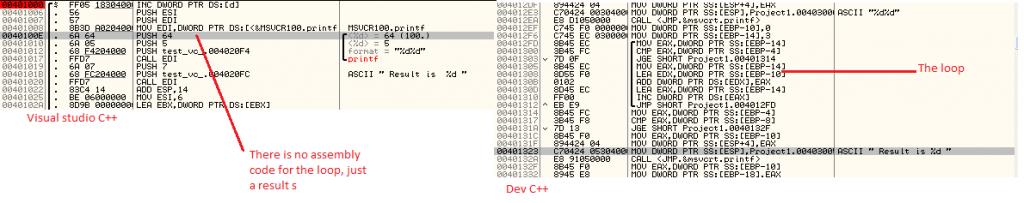I analyzed some simple source code examples running in visual C++ (without optimization option) and Dev C++. I saw that VC++ code is slightly optimized then DEV C++. The following code shows the difference between function calls on both the compilers.

 In visual C++: push eax mov ecx, [local.1] push ecx call F Dev C++: mov DWORD PTR SS:[esp+4], eax mov ecx, [local.1] mov DWORD PTR SS:[esp], ecx call F Argument 1   Argument 2

We can see that VC++ code is optimized and using PUSH instruction instead of MOV

The difference when turning on optimization option:

I also analyzed some codes by turning on the optimization options, I found very interesting results. I compiled the below code on both the compilers to see the effect of optimization.

```int s;
printf("%d%d",a,c);
s=0;

for (int i=3; i< a; i++)
{
s=s+i;
}

if (a <b)
printf( " Result is %d",s);```

Compiled code:As you can see in the images that in VC++ results were calculated at the time of compilation, the for loop result is hardcoded into the binary while in DEV C++ we can see the actual for loop which will calculate the value(result) during run time. However from debugging perspective the DEV C++ code is easy to debug because its structure is similar to the source code, Often times optimized code is mangled and not easy to debug.

http://msdn.microsoft.com/en-us/library/8f8h5cxt.aspx

http://msdn.microsoft.com/en-us/library/ms241594(v=vs.80).aspx

In general, optimization is used for following purposes:

–       Variables are kept in register as long as possible in order to reduce burden of reading/writing from/to memory, increasing processing speed and reducing size of codes

–       JIT optimization